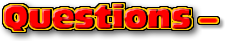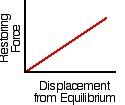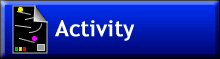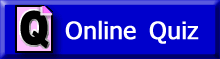This text is meant to accompany class discussions. It is not everything there is to know about simple harmonic motion. It is meant as a  prep for class.Click for the questions that go with this reading
Contents
 Page Description 0 Objectives 1 Hooke's Law 2 Type's of Oscillations 3 Springs 4 Pendulums
Objectives

Students will be able to:

• Students should be able to:

• List the three basic types of harmonic motion and give an example of each.

• Describe the properties that affect the period of motion for an object exhibiting simple harmonic motion.

• Be able to define all the vocaulary words and phrases associated wtih the unit. They include, but they are not limited to; frequency, period, amplitude, displacement from equilibrium, natural frequency, resonant frequency, bob, force constant, spring constant, Hz, cps, RPM and motion cycle.

• Identify the beginning and ending points for the pendulum arm’s length.

• Identify the name of the mass on the end of a pendulum.

• Describe what supplies the “restoring force” for a pendulum.

• Mathematically describe the effect of a pendulum’s mass on the period of motion.

• Mathematically describe the effect of the distance a pendulum is pulled back from its equilibrium position on the period of motion.

• Identify the variables used in the simple harmonic motion problems.

• Solve mathematical problems involving a pendulum’s harmonic motion’s formula.

• Solve pendulum problems utilizing ratio methods.

• Write the equation for a spring’s restoring force while identifying what each variable stands for and its units.

• Use a graph of restoring force versus displacement from equilibrium to calculate a spring force constant.
•• Solve mathematical problems involving a spring’s restoring force formula.

• Mathematically describe the effect of the distance, a mass attached to a spring’s end, is pulled back from its equilibrium position on the period of motion.

• Solve mathematical problems involving a pendulum’s harmonic motion’s formula.

• Solve spring problems utilizing ratio methods.Some solutions to workbook problemsHooke's Law virtual lab, http://goo.gl/8pacv9Review Quiz for pendulumsReveiw quiz for springsMastery Assignment (pdf)Teachers, I created and use these animations in class in PowerPoint slide. They drag them in like a picture. They play like movies. Some I reference and use via a web browser.

by Tony Wayne ...(If you are a teacher, please feel free to use these resources in your teaching.)

 The owner of this website does not collect cookies when the site is visited. However, this site uses and or embeds Adobe, Apple, GoDaddy, Google, and YouTube products. These companies collect cookies when their producs are used on my pages. Click here to go to them to find out more about how they use their cookies. If you do not agree with any of their policies then leave this site now.